#### 联系我们

• 电话：0517-86933868
• 电话：0517-86933865
• 电话：0517-86933866
• 节假日商务联系电话：
• 何经理 18352329759
• 刘经理 18352329763
• 传真：0517-86933869
• E-mail：jszhckyb@163.com
• 公司网址：http://www.zhckyb.com/
• 公司地址：江苏省金湖县工业园区环城西路269号

# 热电偶偏差热电势曲线拟合的研究

1测量模型
热电偶的国际温标ITS-90由NIST美国国家标准与技术研究院在1993年发布热电偶的参考热电势计算公式为:公式中Eref为ITS-90温标的标准参考热电势值△E为偏差热电势值。△E的数值如何确定直接关系到热电偶传感器在任意温度点的热电势求解。同(R型热电偶内插公式的分析与研究》论文所述-样建立偏差热电势与温度的拟合公式测量模型。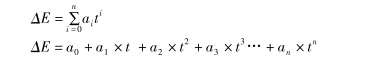依据表1在6个固定点有6个热电势插值与之对应。研究过程就是需要对这些离散的数据点集进行拟合使得拟合的曲线尽量多的穿过所给出的离散点并且.误差值尽可能小。从而通过拟合的函数找出离散点的计算规律，以此推断出任意温度点的热电势偏差值。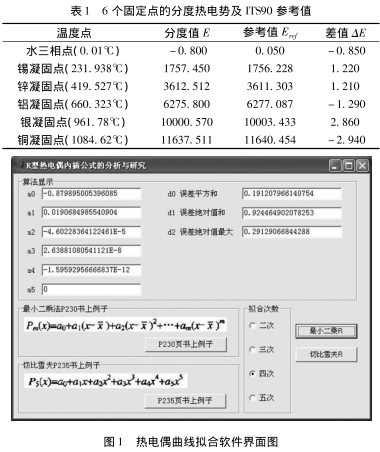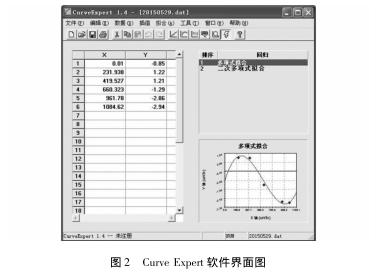2拟合计算
最小二乘法和切比雪夫拟合算法计算针对20阶以内的数据拟合有效对于热电偶的数据拟合而言,已经足.以满足计算要求标定的数据点一般为6个,曲线拟合n值为1至5。
2.1最小二乘法
n=5时课题拟合计算结果如下但是CurveExpert软件提示6个数据点并不满足5次的曲线拟合要求,因此不采用此组拟合曲线参数。
a0=-0.850041998463831
al=0.00419920929112408
a2=6.37116245722718E-5
a3=-2.44436511901853E-7
a4=2.70582418257385E-10
a5=-9.60481199754648E-14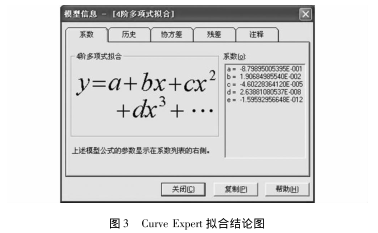n=4时拟合曲线的系数如下所示与图3所示的CurveExpert拟合系数对比验证系数完全一致达到预期计算效果同样n为3和2时的计算系数经验证也完全一致。
a0=-0.879895005396085
al=0.0190684985540904
a2=-4.60228364122461E-5
a3=2.63881080541121E-8
a4=-1.59592956666837E-12n=3时
a0=-0.874781128095732
al=0.0186127951094208
a2=-4.37481584610491E-5
a3=.2.29522241448483E-8
n=2时
a0=-0.27389292847006
al=0.00486367370529746
a2=-7.29961208590609E-6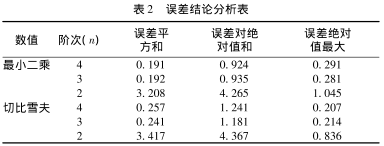3.2切比雪夫法
切比雪夫曲线拟合的系数如下
n=5时
a0=-I.0570321937135
al=0.020123509226792
a2=-4.57805634220434E-5
a3=2.19960749682939E-8
a4=1.65323650831879E-12
a5=0.206830963199262
n=4时
a0=-1.0570321937135
al=0.020123509226792
a2=-4.57805634220434E-5
a3=2.19960749682939E-8
a4=.1.65323650831879E-12
n=3时
a0=-1.06379901987901
al=0.0204501360801971
a2=-4.75657684751315E-5
a3=2.50891732556674E-8
n=2时
a0=-0.0138754403562611
al=0.00369513844519069
a2=-6.60498430353681E-6
3结论
将上述两种方法求得的拟合曲线分别带入6个固定点数值得出表2所示的误差结论分析表。
为了便于查看保留3位有效数字很容易的可以看出最小二乘法n=4时6个固定点的误差平方和最小;切比雪夫法n=4时6个固定点的误差绝对值最大值最小。阶次越大曲线拟合的效果越好越能满足热电偶在不同温度点的热电势偏差值计算要求。